International
Tables for
Crystallography
Volume A
Space-group symmetry
Edited by Th. Hahn

International Tables for Crystallography (2006). Vol. A, ch. 1.1, p. 2

## Section 1.1.1. Vectors, coefficients and coordinates

Th. Hahna*

aInstitut für Kristallographie, Rheinisch-Westfälische Technische Hochschule, Aachen, Germany
Correspondence e-mail: hahn@xtal.rwth-aachen.de

### 1.1.1. Vectors, coefficients and coordinates

| top | pdf |

Printed symbolExplanation
a, b, c; or ai Basis vectors of the direct lattice
a, b, c Lengths of basis vectors, lengths of cell edges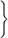Lattice or cell parameters
α, β, γ Interaxial (lattice) angles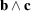,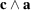,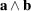V Cell volume of the direct lattice
G Matrix of the geometrical coefficients (metric tensor) of the direct lattice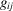Element of metric matrix (tensor) G
r; or x Position vector (of a point or an atom)
r Length of the position vector r
xa, yb, zc Components of the position vector r
x, y, z; or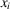Coordinates of a point (location of an atom) expressed in units of a, b, c; coordinates of end point of position vector r; coefficients of position vector r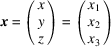Column of point coordinates or vector coefficients
t Translation vector
t Length of the translation vector t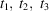; orCoefficients of translation vector t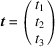Column of coefficients of translation vector t
u Vector with integral coefficients
u, v, w; or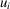Integers, coordinates of a (primitive) lattice point; coefficients of vector u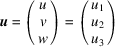Column of integral point coordinates or vector coefficients
o Zero vector
o Column of zero coefficients
a′, b′, c′; or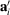New basis vectors after a transformation of the coordinate system (basis transformation)
r′; or x′; x′, y′, z′; or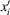Position vector and point coordinates after a transformation of the coordinate system (basis transformation); or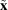;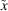,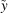,; or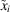New position vector and point coordinates after a symmetry operation (motion)